NCERT Solutions for Class 6 Maths Chapter 12 Ratio and Proportion Ex 12.1 are part of NCERT Solutions for Class 6 Maths. Here we have given NCERT Solutions for Class 6 Maths Chapter 12 Ratio and Proportion Ex 12.1.

 Board CBSE Textbook NCERT Class Class 6 Subject Maths Chapter Chapter 12 Chapter Name Ratio and Proportion Exercise Ex 12.1 Number of Questions Solved 16 Category NCERT Solutions

## NCERT Solutions for Class 6 Maths Chapter 12 Ratio and Proportion Ex 12.1

Question 1.
There are 20 girls and 15 boys in a class.
(a) What is the ratio of a number of girls to the number of boys?
(b) What is the ratio of a number of girls to the total number of students in the class?
Solution :
(a) Ratio of number of girls to the number of boys(b) Total number of students in the class = 20 + 15 = 35
∴ Ratio of number of girls to the total number of students in the classQuestion 2.
Out of 30 students in a class, 6 like football, 12 like cricket and remaining like tennis. Find the ratio of:
(a) Number of students liking football to a number of students liking tennis.
(b) Number of students liking cricket to the total number of students.
Solution :
(a) Number of students liking tennis = 30-(6+ 12) = 30 – 18 = 12
∴ Ratio of number of students liking football to number of students liking tennis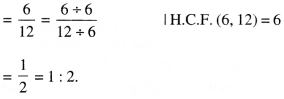(b) Number of students liking cricket to total number of students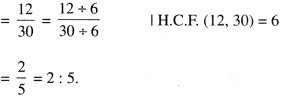Question 3.
See the figure and find the ratio of:
(a) Number of triangles to the number of circles inside the rectangle.(b) Number of squares to all the figures inside the rectangle.
(c) Number of circles to all the figures inside the rectangle.
Solution :
(a) Number of triangle inside the rectangle = 3 . , Number of circles inside the rectangle = 2 Ratio of number of triangles to the number of circles inside the rectangle = $$\frac{ 3 }{ 2 }$$ =3:2.
(b) Number of squares inside the rectangle = 2 Number of all the figures inside the rectangle = 7
∴ Ratio of number of squares to all the figures 2
inside the rectangle = $$\frac{ 2 }{ 7 }$$ =2:7.
(c) Ratio of number of circles to all the figures inside the rectangle = $$\frac{ 2 }{ 7 }$$ =2:1.

Question 4.
Distance travelled by Hamid and Akhtar in an hour are 9 km and 12 km. Find the ratio of speed of Hamid to the speed of Akhtar.
Solution :
Ratio of speed of Hamid to the speed ofQuestion 5.
Fill in the following blanks: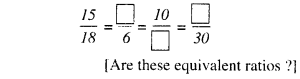Solution :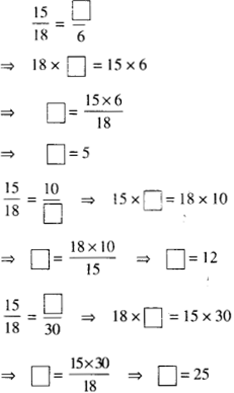Question 6.
Find the ratio of the following :
(a) 81 to 108
(b) 98 to 63
(c) 33 km to 121 km
(d) 30 minutes to 45 minutes.
Solution :Question 7.
Find the ratio of the following :
(a) 30 minutes to 1.5 hours
(b) 40 cm to 1.5 m
(c) 55 paise to ₹ 1
(d) 500 ml to 2 litres.
Solution :
(a) 1.5 hours = 1.5 × 60 minutes = 90 minutes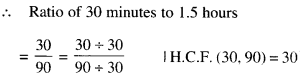Question 8.
In a year, Seema earns- ₹ 1,50.000 and saves ₹ 50,000. Find the ratio of:
(a) Money that Seema earns to the money she saves.
(b) Money that she saves to the money that she spends.
Solution :
(a) Ratio of money that Seema earns to the money she saves(b) Money that she spends
= ₹ 1,50,000 – ₹ 50,000 = ₹ 1,00,000
∴ Ratio of money she saves to the money she spends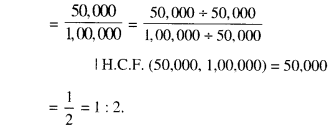Question 9.
There are 102 teachers in a school of 3300 students. Find the ratio of the number of teachers to the number of students.
Solution :
Ratio of the number of teachers to the number of students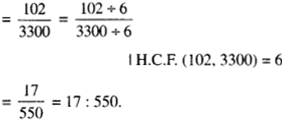Question 10.
In a college, out of4320 students, 2300 are girls. Find the ratio of:
(a) Number of girls to the total number of students.
(b) Number of boys to the number of girls.
(c) Number of boys to the total number of students.
Solution :
(a) Ratio of number of girls to the total number of students(b) Number of boys = 4320 – 2300 = 2020
∴ Ratio of number of boys to the number of girl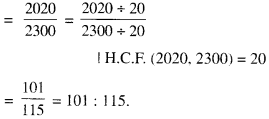(c) Ratio of number of boys to the total number of students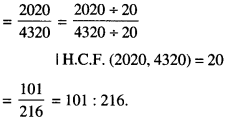Question 11.
Out of 1800 students in a school, 750 opted basketball, 800 opted cricket and remaining opted table tennis. If a student can opt only one game, find the ratio of:
(a) Number of students who opted basketball to the number of students who opted table tennis.
(b) Number of students who opted cricket to the number of students opting basketball.
(c) Number of students who opted basketball to the total number of students.
Solution :
(a) Number of students opting table tennis = 1800 – (750 + 800) = 1800 -1550 = 250
∴ Ratio of number of students opting basketball to number of students opting table tennis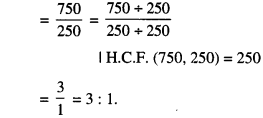(b) Ratio of number of students opting cricket to the number of students opting basketball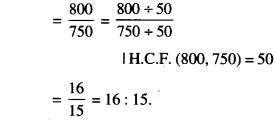(c) Ratio of number of students opting basketball to the total number of students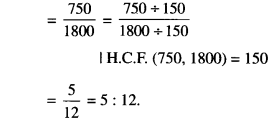Question 12.
Cost of a dozen pens is ₹ 180 and cost of 8 ball pens is ₹ 56. Find the ratio of cost of a pen to the cost of a ball pen.
Solution :
1 dozen =12 items
∴ Cost of 12 pens = ₹ 180
∴ Cost of 1 pen = ₹ $$\frac{ 180 }{ 12 }$$ = ₹ 15
∴ Cost of 8 ball pens = ₹ 56
∴ Cost of 1 ball pen = ₹ $$\frac{ 56 }{ 8 }$$ = ₹ 7
∴ Ratio of cost of a pen to the cost of a ball 15 pen = $$\frac{ 15 }{ 7 }$$ =15:7.

Question 13.
Consider the statement: Ratio of breadth and length of a hall 2: 5. Complete the following table that shows some possible breadths and lengths of the hall.Solution :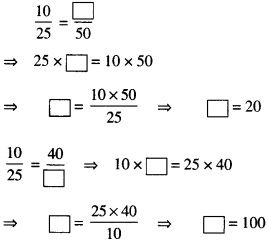Hence, the completed table is as follows:Question 14.
Divide 20 pens between Sheela and Sangeeta in the ratio 3: 2.
Solution :
Total number of pens = 20 Ratio = 3:2
Sum of the parts = 3 + 2 = 5
∴ Sheel’s shae = $$\frac{ 3 }{ 5 }$$ × 20 = 12 and, Samgeeta’s share = $$\frac{ 2 }{ 5 }$$ × 20 = 8

Question 15.
Mother wants to divide ₹ 36 between her daughters Shreya and Bhoomika in the ratio of their ages. If the age of Shreya is 15 years and age of Bhoomika is 12 years, find how much Shreya and Bhoomika will get?
Solution :
Total money = ₹ 36
The ratio of the ages of Shreya and Bhoomika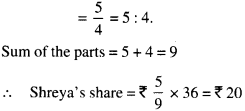Hence, Shreya will get ₹ 20 and Bhoomika will get ₹ 16.

Question 16.
Present age of the father is 42 years and that of his son is 14 years. Find the ratio of :
(a) Present age of father to the present age of the son.
(b) Age of the father to the age of son, when the son was 12 years old.
(c) Age of father after 10 years to the age of son after 10 years.
(d) Age of father to the age of son when father was 30 years old.
Solution :
(a) Ratio of the present age of father to the present age of the son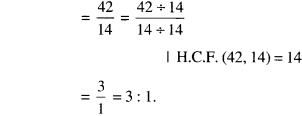(b) Son was 12 years old 14 – 12 = 2 years before
Age of the father 2 years before = 42 – 2 = 40 years
∴ Ratio of the age of the father to the age of the son. when son was 12 years old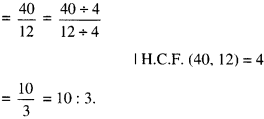(c) Age of father after 10 years = 42 + 10 = 52 years
Age of son after 10 years = 14 + 10 = 24 years
∴ Ratio of age of father after 10 years to the age of son after 10 years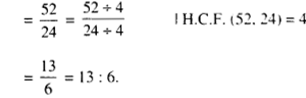(d) Father was 30 years old 42 – 30 = 12 years before
Age of son 12 years before = 14 – 12 = 2 years
∴ Ratio of the age of father to the age of son when father was 30 years old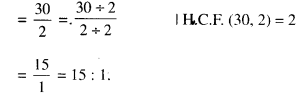We hope the NCERT Solutions for Class 6 Maths Chapter 12 Ratio and Proportion Ex 12.1 help you. If you have any query regarding NCERT Solutions for Class 6 Maths Chapter 12 Ratio and Proportion Ex 12.1, drop a comment below and we will get back to you at the earliest.